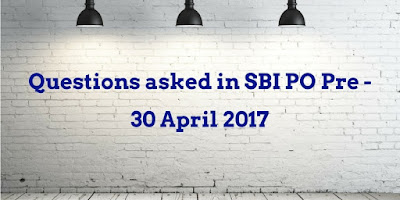# Questions asked in SBI PO Prelims - 30 April 2017Hi students,
I am sharing questions asked in Quantitative Aptitude section of today's SBI PO Prelims exam. Similar questions will be asked in upcoming slots.

Questions asked in SBI PO Preliminary Exam :-

### Miscellaneous Questions -

1. A, B and C started a business where their initial capital was in the ratio of 2:3:4. At the end of 6 months, A invested an amount such that his initial capital became equal to B's initial capital investment. If the annual profit of B is Rs. 3000 then what is the total profit?

2. A dealer sells two A and B article at 20% and 30% profit. Total profit earned by him is 140. Cost price of article A is 50 less than cost price if article B. Find the cp of B.

3. Time taken by A alone to finish a work is 50% more than the time taken by A and B together. B is thrice as efficient as C. If B and C together can complete the work in 22.5 days, then how many days will A alone take to complete the work?

4. A vessel contains milk and water in the ratio of 7:1. 1/10th mixture is removed and 3 litres of water is added. The final ratio of milk and water is 6:1. Find the initial quantity of the mixture.

5. Suman spends 36% on food. Remaining ¼ on brother and ⅜ on sister. The difference of amount given to brother and sister is 1200. What is suman's total savings ?

### Number Series (1st Slot) -

1. 9 8 14 39 ? 755
2. 25 32 21 34 17 ?
3. 11 6 7 12 ? 67.5
4. 4 7 35 83 146 ?
5. 521 496 447 366 245

X² = 81

Y² – 22y + 121 = 0#### What's trending in BankExamsToday

Smart Prep Kit for Banking Exams by Ramandeep Singh - Download here